Algebraic Geometry Commons™

223 Full-Text Articles 268 Authors 56,862 Downloads69 Institutions

All Articles in Algebraic Geometry

223 full-text articles. Page 5 of 9.

A Study Of Green’S Relations On Algebraic Semigroups, 2015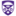The University of Western Ontario

A Study Of Green’S Relations On Algebraic Semigroups, Allen O'Hara

Electronic Thesis and Dissertation Repository

The purpose of this work is to enhance the understanding regular algebraic semigroups by considering the structural influence of Green's relations. There will be three chief topics of discussion.

• Green's relations and the Adherence order on reductive monoids
• Renner’s conjecture on regular irreducible semigroups with zero
• a Green’s relation inspired construction of regular algebraic semigroups

Primarily, we will explore the combinatorial and geometric nature of reductive monoids with zero. Such monoids have a decomposition in terms of a Borel subgroup, called the Bruhat decomposition, which produces a finite monoid, R, the Renner monoid. We will explore ...

Algorithms To Compute Characteristic Classes, 2015The University of Western Ontario

Algorithms To Compute Characteristic Classes, Martin Helmer

Electronic Thesis and Dissertation Repository

In this thesis we develop several new algorithms to compute characteristics classes in a variety of settings. In addition to algorithms for the computation of the Euler characteristic, a classical topological invariant, we also give algorithms to compute the Segre class and Chern-Schwartz-MacPherson (CSM) class. These invariants can in turn be used to compute other common invariants such as the Chern-Fulton class (or the Chern class in smooth cases).

We begin with subschemes of a projective space over an algebraically closed field of characteristic zero. In this setting we give effective algorithms to compute the CSM class, Segre class and ...

Dihedral-Like Constructions Of Automorphic Loops, 2015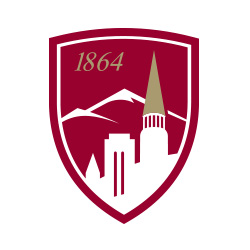University of Denver

Dihedral-Like Constructions Of Automorphic Loops, Mouna Ramadan Aboras

Electronic Theses and Dissertations

In this dissertation we study dihedral-like constructions of automorphic loops. Automorphic loops are loops in which all inner mappings are automorphisms. We start by describing a generalization of the dihedral construction for groups. Namely, if (G , +) is an abelian group, m > 1 and α ∈2 Aut(G ), let Dih(m, G, α) on Zm × G be defined by

(i, u )(j, v ) = (i + j , ((-1)j u + vij ).

We prove that the resulting loop is automorphic if and only if m = 2 or (α2 = 1 and m is even) or (m is odd, α = 1 and ...

Symbolic Powers Of Edge Ideals, 2015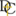Dordt College

Symbolic Powers Of Edge Ideals, Mike Janssen

Faculty Work Comprehensive List

No abstract provided.

Manipulating The Mass Distribution Of A Golf Putter, 2015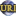University of Rhode Island

Manipulating The Mass Distribution Of A Golf Putter, Paul J. Hessler Jr.

Senior Honors Projects

Putting may appear to be the easiest but is actually the most technically challenging part of the game of golf. The ideal putting stroke will remain parallel to its desired trajectory both in the reverse and forward direction when the putter head is within six inches of the ball. Deviation from this concept will cause a cut or sidespin on the ball that will affect the path the ball will travel.

Club design plays a large part in how well a player will be able to achieve a straight back and straight through club head path near impact; specifically the ...

Automorphisms Of Graph Curves On K3 Surfaces, 2015Georgia Southern University

Automorphisms Of Graph Curves On K3 Surfaces, Joshua C. Ferrerra

Electronic Theses and Dissertations

We examine the automorphism group of configurations of rational curves on \$K3\$ surfaces. We use the properties of finite automorphisms of \$\PP^1\$ to examine what restrictions a given elliptic fibration imposes on the possible finite order non-symplectic automorphisms of the \$K3\$ surface. We also examine the fixed loci of these automorphisms, and construct an explicit fibration to demonstrate the process.

2014The University of Western Ontario

Computing Intersection Multiplicity Via Triangular Decomposition, Paul Vrbik

Electronic Thesis and Dissertation Repository

Fulton’s algorithm is used to calculate the intersection multiplicity of two plane curves about a rational point. This work extends Fulton’s algorithm first to algebraic points (encoded by triangular sets) and then, with some generic assumptions, to l many hypersurfaces.

Out of necessity, we give a standard-basis free method (i.e. practically efficient method) for calculating tangent cones at points on curves.

Continuous Dependence Of Solutions Of Equations On Parameters, 2014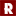Rose-Hulman Institute of Technology

Continuous Dependence Of Solutions Of Equations On Parameters, Sean A. Broughton

Mathematical Sciences Technical Reports (MSTR)

It is shown under very general conditions that the solutions of equations depend continuously on the coefficients or parameters of the equations. The standard examples are solutions of monic polynomial equations and the eigenvalues of a matrix. However, the proof methods apply to any finite map T : Cn -> Cn.

2014The University of Western Ontario

Tilting Sheaves On Brauer-Severi Schemes And Arithmetic Toric Varieties, Youlong Yan

Electronic Thesis and Dissertation Repository

The derived category of coherent sheaves on a smooth projective variety is an important object of study in algebraic geometry. One important device relevant for this study is the notion of tilting sheaf.

This thesis is concerned with the existence of tilting sheaves on some smooth projective varieties. The main technique we use in this thesis is Galois descent theory. We first construct tilting bundles on general Brauer-Severi varieties. Our main result shows the existence of tilting bundles on some Brauer-Severi schemes. As an application, we prove that there are tilting bundles on an arithmetic toric variety whose toric variety ...

Light Pollution Research Through Citizen Science, 2014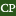CSU Sacramento

Light Pollution Research Through Citizen Science, John Kanemoto

STAR (STEM Teacher and Researcher) Presentations

Light pollution (LP) can disrupt and/or degrade the health of all living things, as well as, their environments. The goal of my research at the NOAO was to check the accuracy of the citizen science LP reporting systems entitled: Globe at Night (GaN), Dark Sky Meter (DSM), and Loss of the Night (LoN). On the GaN webpage, the darkness of the night sky (DotNS) is reported by selecting a magnitude chart. Each magnitude chart has a different density/number of stars around a specific constellation. The greater number of stars implies a darker night sky. Within the DSM iPhone ...

2014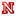University of Nebraska-Lincoln

The Neural Ring: Using Algebraic Geometry To Analyze Neural Codes, Nora Youngs

Dissertations, Theses, and Student Research Papers in Mathematics

Neurons in the brain represent external stimuli via neural codes. These codes often arise from stimulus-response maps, associating to each neuron a convex receptive field. An important problem confronted by the brain is to infer properties of a represented stimulus space without knowledge of the receptive fields, using only the intrinsic structure of the neural code. How does the brain do this? To address this question, it is important to determine what stimulus space features can - in principle - be extracted from neural codes. This motivates us to define the neural ring and a related neural ideal, algebraic objects that encode ...

A Kleinian Approach To Fundamental Regions, 2014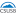Joshua L Hidalgo

A Kleinian Approach To Fundamental Regions, Joshua L. Hidalgo

Electronic Theses, Projects, and Dissertations

This thesis takes a Kleinian approach to hyperbolic geometry in order to illustrate the importance of discrete subgroups and their fundamental domains (fundamental regions). A brief history of Euclids Parallel Postulate and its relation to the discovery of hyperbolic geometry be given first. We will explore two models of hyperbolic \$n\$-space: \$U^n\$ and \$B^n\$. Points, lines, distances, and spheres of these two models will be defined and examples in \$U^2\$, \$U^3\$, and \$B^2\$ will be given. We will then discuss the isometries of \$U^n\$ and \$B^n\$. These isometries, known as M\"obius ...

The Dual Gromov-Hausdorff Propinquity, 2014University of Denver

The Dual Gromov-Hausdorff Propinquity, Frédéric Latrémolière

Mathematics Preprint Series

Motivated by the quest for an analogue of the Gromov-Hausdorff distance in noncommutative geometry which is well-behaved with respect to C*- algebraic structures, we propose a complete metric on the class of Leibniz quantum compact metric spaces, named the dual Gromov-Hausdorff propinquity, which resolves several important issues raised by recent research in noncommutative metric geometry: our new metric makes *-isomorphism a necessary condition for distance zero, is well-adapted to Leibniz seminorms, and — very importantly — is complete, unlike the quantum propinquity which we introduced earlier. Thus our new metric provides a new tool for noncommutative metric geometry which offers a solution ...

A Metric On Max-Min Algebra, 2014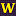University of Oregon

A Metric On Max-Min Algebra, Jonathan Eskeldson, Miriam Jaffe, Viorel Nitica

Mathematics

No abstract provided.

Tropical Convexity Over Max-Min Semiring, 2014West Chester University of Pennsylvania

Tropical Convexity Over Max-Min Semiring, Viorel Nitica, Sergei Sergeev

Mathematics

No abstract provided.

Early Investigations In Conformal And Differential Geometry, 2014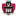University of Arkansas, Fayetteville

Early Investigations In Conformal And Differential Geometry, Raymond T. Walter

Inquiry: The University of Arkansas Undergraduate Research Journal

The present article introduces fundamental notions of conformal and differential geometry, especially where such notions are useful in mathematical physics applications. Its primary achievement is a nontraditional proof of the classic result of Liouville that the only conformal transformations in Euclidean space of dimension greater than two are Möbius transformations. The proof is nontraditional in the sense that it uses the standard Dirac operator on Euclidean space and is based on a representation of Möbius transformations using 2x2 matrices over a Clifford algebra. Clifford algebras and the Dirac operator are important in other applications of pure mathematics and mathematical physics ...

2014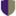Harvey Mudd College

Arithmetical Graphs, Riemann-Roch Structure For Lattices, And The Frobenius Number Problem, Jeremy Usatine

HMC Senior Theses

If R is a list of positive integers with greatest common denominator equal to 1, calculating the Frobenius number of R is in general NP-hard. Dino Lorenzini defines the arithmetical graph, which naturally arises in arithmetic geometry, and a notion of genus, the g-number, that in specific cases coincides with the Frobenius number of R. A result of Dino Lorenzini's gives a method for quickly calculating upper bounds for the g-number of arithmetical graphs. We discuss the arithmetic geometry related to arithmetical graphs and present an example of an arithmetical graph that arises in this context. We also discuss ...

Function Of Several Variable, 2014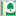Parkland College

Function Of Several Variable, Weiting Li

A with Honors Projects

This Parkland A with Honors project discusses the function of several variables, it's limits and partial derivatives.

2013University of Nebraska-Lincoln

Geometric Study Of The Category Of Matrix Factorizations, Xuan Yu

Dissertations, Theses, and Student Research Papers in Mathematics

We study the geometry of matrix factorizations in this dissertation.
It contains two parts. The first one is a Chern-Weil style
construction for the Chern character of matrix factorizations; this
allows us to reproduce the Chern character in an explicit,
understandable way. Some basic properties of the Chern character are
also proved (via this construction) such as functoriality and that
it determines a ring homomorphism from the Grothendieck group of
matrix factorizations to its Hochschild homology. The second part is
a reconstruction theorem of hypersurface singularities. This is
given by applying a slightly modified version of Balmer's tensor
triangular ...

2013University of Nebraska-Lincoln

Results On Containments And Resurgences, With A Focus On Ideals Of Points In The Plane, Annika Denkert

Dissertations, Theses, and Student Research Papers in Mathematics

Let K be an algebraically closed field and IR=K[PN] a nontrivial homogeneous ideal. We can describe ordinary powers Ir and symbolic powers I(m) of I. One question that has been of interest over the past couple of years is that of when we have containment of I(m) in Ir. Bocci and Harbourne defined the resurgence of I as rho(I)=supm,r{m/r | I(m) is not contained in Ir}. Hence in particular I(m)Ir whenever m/r is at least rho(I). Results by Macaulay, Ein-Lazarsfeld-Smith ...